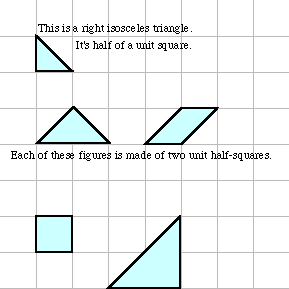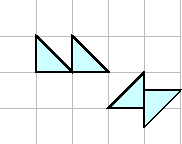www.MathEd.page/puzzles
Adapted from Geometry Labs, by Henri Picciotto

# Discovering the SuperTangramsHow many unit half-squares in these? _____ and _____

Note: arrangements like these do not count. The unit half-squares much touch by whole sides.1. There are only four figures that are made of three unit half-squares. Use graph paper to find them.
2. Figures that are made up of four unit half-squares are called superTangrams. How many of them can you find?
3. Find the perimeters of the superTangrams, and rank them from shortest to longest.

## Discussion

1. What is your method for finding superTangram shapes?
2. How do you know when you have found all the superTangrams?